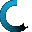Retrieving data ...
Please do not click anywhere else.

If you see no response after 2-3 minutes, then click on the [X] button in the upper right corner to close the window and retry the operation at a later time.
Interactive Multimedia Learning & Teaching Management System Suite## Course Contents

• 1. Basic Number Sense
•  1.1 The Numbering System and Place Value
•  1.2 Ways to Name Numbers
•  1.3 Number Forms
•  1.4 Defining and Grouping Whole Numbers
•  1.5 Reading and Writing Whole Numbers
•  1.6 Number Combinations
•  1.7 Comparing Numbers
•  1.8 Ordering Numbers
•  1.9 Even and Odd Numbers
•  1.10 Rounding Numbers
•  1.11 Word Problems
•  2.3 Adding a 2-digit Number and a 2-digit Number
•  2.4 Adding a 3-digit Number and a 2-digit Number
•  2.5 Adding a 4-digit Number and a 2-digit Number
•  2.6 Adding a 5-digit Number and a 2-digit Number
•  2.7 Adding a 3-digit Number and a 3-digit Number
•  2.8 Adding a 4-digit Number and a 3-digit Number
•  2.9 Adding a 5-digit Number and a 3-digit Number
•  2.10 Adding a 5-digit Number and a 4-digit Number
•  2.11 Estimating a Sum by Rounding
•  2.12 Adding 3 Number Sets
• 3. Subtraction
•  3.1 Review of Subtraction
•  3.2 Subtraction in Relation to Addition
•  3.3 Properties of Subtraction
•  3.4 Subtracting a 1-digit Number from a 2-digit Number
•  3.5 Subtraction Involving Borrowed Numbers
•  3.6 Subtracting a 2-digit Number from a 2-digit Number
•  3.7 Subtracting a 2-digit Number from a 3-digit Number
•  3.8 Subtracting a 2-digit Number from a 4-digit Number
•  3.9 Subtracting a 3-digit Number from a 3-digit Number
•  3.10 Subtracting a 3-digit Number from a 4-digit Number
•  3.11 Subtracting a 4-digit Number from a 4-digit Number
•  3.12 Estimating Subtraction with Large Numbers
• 4. Multiplication
•  4.1 Multiplication in Relation to Addition
•  4.2 Properties of Multiplication
•  4.3 Multiplication Table
•  4.4 Multiplication Tricks
•  4.5 Multiplying a 1-digit Number and a 1-digit Number
•  4.6 Multiplying a 2-digit Number and a 1-digit Number
•  4.7 Multiplying a 3-digit Number and a 1-digit Number
•  4.8 Multiplying a 2-digit Number and a 2-digit Number
•  4.9 Multiplying a 3-digit Number and a 2-digit Number
•  4.10 Multiplication with Three Numbers
• 5. Division
•  5.1 Division Facts and Terms
•  5.2 Division in Relation to Subtraction
•  5.3 Division in Relation to Multiplication
•  5.4 Basic Properties of Division
•  5.5 Division Tables
•  5.6 Dividing Using the Division Bracket
•  5.7 Division with a Remainder
•  5.8 Dividing a 1-digit Number by a 1-digit Number
•  5.9 Dividing a 2-digit Number by a 1-digit Number
•  5.10 Dividing a 2-digit Number by a 2-digit Number
•  5.11 Dividing a 3-digit Number by a 1-digit Number
•  5.12 Dividing a 3-digit Number by a 2-digit Number
• 6. Decimal Numbers
•  6.1 Introduction to Decimal Numbers
•  6.2 Displaying Decimal Numbers
•  6.3 Counting Money with Decimals
•  6.5 Subtracting Decimal Numbers
• 7. Fractions
•  7.1 Introduction to Fractions
•  7.2 Identifying Fractions
•  7.3 Equivalent Fractions
•  7.4 Comparing Fractions
•  7.6 Subtracting Fractions
• 8. Math Operations Using a Calculator
•  8.1 Introduction to Calculators
•  8.2 Solving Addition Problems using a Calculator
•  8.3 Adding Large Numbers using a Calculator
•  8.4 Adding Three Sets of Numbers using a Calculator
•  8.5 Adding Decimal Numbers using a Calculator
•  8.6 Solving Subtraction Problems using a Calculator
•  8.7 Subtracting Decimal Numbers using a Calculator
•  8.8 Solving Multiplication Problems using a Calculator
•  8.9 Solving Division Problems using a Calculator
• 9. Basic Graphs
•  9.1 Introduction to Graphs
•  9.2 Circle Graphs
•  9.3 Creating Circle Graphs
•  9.4 Interpreting Circle Graphs
•  9.5 Bar Graphs
•  9.6 Creating Bar Graphs
•  9.7 Interpreting Bar Graphs
• 10. Time and Money
•  10.1 Review of Time
•  10.2 Telling Time
•  10.3 Converting Digital and Analog Time
•  10.4 Converting Time
•  10.5 Adding Different Units of Time
•  10.6 Finding Elapsed Time
•  10.8 Counting Money
•  10.9 Converting Money into Decimal Form
•  10.10 Comparing Money
•  10.11 Making Change
• 11. Measurements
•  11.1 Systems of Measurement
•  11.2 Weight
•  11.3 Temperature
•  11.4 Length
•  11.5 Measuring Length
•  11.6 Perimeter
•  11.7 Area
•  11.8 Volume
• 12. Algebra
•  12.1 Numeric Expressions and Equations
•  12.2 Number Comparisons using Symbols
•  12.3 Completing Number Sentences with Addition
•  12.4 Comparing Number Expressions with Addition
•  12.5 Completing Number Sentences with Subtraction
•  12.6 Comparing Number Expressions with Subtraction
•  12.7 Patterns using Shapes and Symbols
•  12.8 Number Patterns using Addition and Subtraction
•  12.9 Number Patterns using Multiplication and Division
•  12.10 Unknown Variables in Mathematical Equations
•  12.11 The Number Line
• 13. Geometry
•  13.1 Basic Geometry Review
•  13.2 Points, Lines and Line Segments
•  13.3 Measuring Line Segments
•  13.4 Parallel, Intersecting and Perpendicular Lines
•  13.5 Angles
•  13.6 Triangles